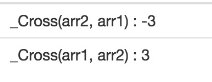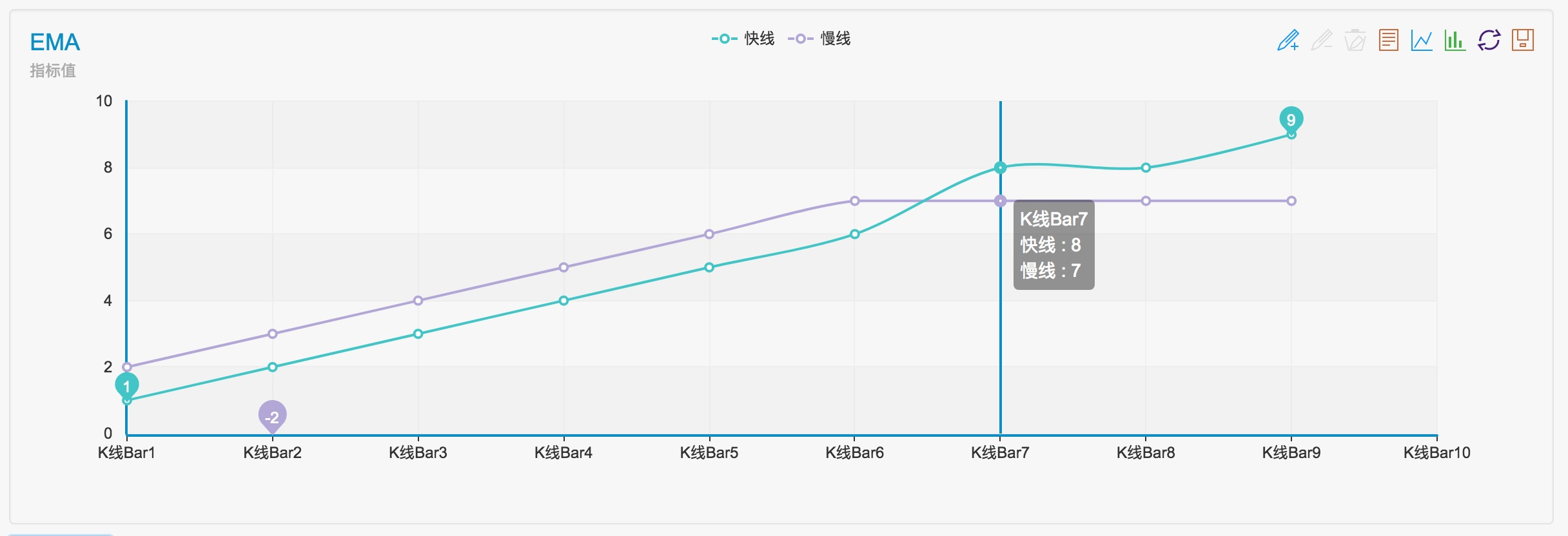# Analysis and instructions for use about built-in function _Cross

Author: Lydia, Created: 2023-10-07 15:35:44, Updated:

## Analysis and instructions for use about built-in function _Cross

The _Cross function in the Global Functions section of the API documentation is used to calculate the crossover state of the two indicators.

### The function implementation is similar to the following code:

Note that when `arr1` is defined as an array of fast indicators and `arr2` as an array of slow indicators, the value returned by the `_Cross` function is positive, i.e., according to the documentation, `a positive number is the period of upward penetration, a negative number indicates the period of downward penetration, and 0 means the same as the current price`. It can be seen, at this time `arr1` upward penetrated the `arr2` has been n cycles, at this time, it’s the fast line upward penetration the slow line, representing a crossup. The same `_Cross` function returns a negative number, that is, a crossdown.

The opposite is true if `arr1` is defined as an array of slow indicators and `arr2` as an array of fast indicators. A positive value returned by the `_Cross` function represents a crossdown. A negative value returned by the `_Cross` function represents a crossup.

``````// Return to the number of periods of upward penetration, the positive number is the number of periods of upward penetration, the negative number indicates the number of periods of downward penetration, 0 means the same as the current price
\$.Cross = function(arr1, arr2) {            // The number of parameters is 2, it can be seen from the parameter name, these two parameters should be an array type, the array is like an array in the X-axis for the array index value, Y-axis for the index value of the line in the coordinate system, the function is to determine the intersection of the two lines
if (arr1.length !== arr2.length) {      // The first step is to determine if the two arrays being compared are equal in length
throw "array length not equal";     // Throw an error if they are not equal, it is not possible to determine the intersection of unequal lines.
}
var n = 0;                              // Declare the variable n to record the intersection state, initially 0, unintersected
for (var i = arr1.length-1; i >= 0; i--) {      // Iterate over the array arr1, from the last element forward.
if (typeof(arr1[i]) !== 'number' || typeof(arr2[i]) !== 'number') { // When any of the arrays arr1 or arr2 is of a non-numeric type (i.e., an invalid indicator), the traversal loop is broken.
break;                                  // break out of a loop
}
if (arr1[i] < arr2[i]) {                    // If arr1 is smaller than arr2 then n--, will record the relative state of arr1, arr2 at the beginning, (i.e., at the beginning n will adjust itself according to the relative sizes of arr1[i], arr2[i], and once there is another relationship between the sizes of arr1[i], arr2[i] opposite to the state of n, i.e., a crossing of the two lines has occurred.)
if (n > 0) {
break;
}
n--;
} else if (arr1[i] > arr2[i]) {             // If arr1 is greater than arr2 then n++
if (n < 0) {
break;
}
n++;
} else {                                    // arr1[i] == arr2[i], then immediately break
break;
}
}
return n;                                       // Returns the value of n, which represents the number of periods that have been crossed, and 0, which means that the indicator values are equal.
};
``````

### Let’s simulate a set of data passed into this parameter and see how it turns out

``````var arr1 = [1,2,3,4,5,6,8,8,9]     // fast line indicator
var arr2 = [2,3,4,5,6,7,7,7,7]     // slow line indicator
function main(){
Log("_Cross(arr1, arr2) : ", _Cross(arr1, arr2))
Log("_Cross(arr2, arr1) : ", _Cross(arr2, arr1))
}
``````We can see the results are 3, -3.As you we see in the chart, the crossover occurs in front of three K-line bars.

More